Here we use quickpsy to illustrate the problem of fitting psychometric functions when lapses of the participants occur. This example is included in the quickpsy paper (Linares & López-Molinerm 2016).

## Lapses can bias threshold estimation

Wichmann and Hill (2001) propose that when lapses occur and are not taken into account in the fitting process, the estimated threshold could be biased. Consider the following example

library(quickpsy)
library(dplyr)
library(ggplot2)

x <- seq(0, 420, 60)
k <- c(0, 0, 4, 18, 20, 20, 19, 20)
dat <- tibble(x, k, n = 20)

fitWithoutLapses <- quickpsy(dat, x, k, n, prob = .75, bootstrap = "none")

curvesWithoutLapses <- fitWithoutLapses$curves %>% mutate(cond = 'Without Lapses') pWithout <- ggplot()+ geom_point(data = fitWithoutLapses$averages, aes(x = x, y = prob)) +
geom_line(data = curvesWithoutLapses,
aes(x = x, y = y, color = cond)) +
geom_linerange(data = fitWithoutLapses$thresholds, aes(x = thre, ymin = 0, ymax = prob), lty =2) pWithout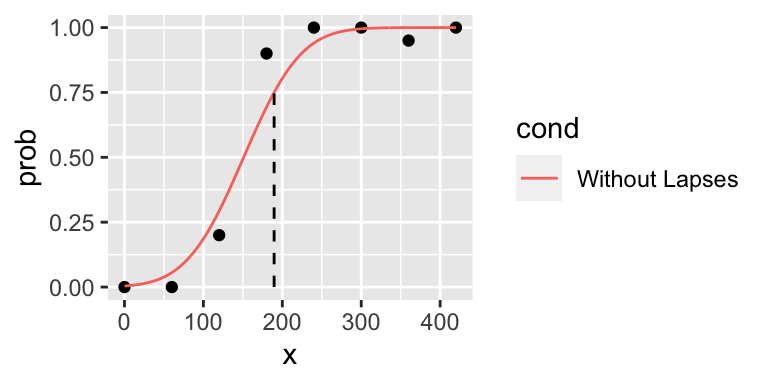In the presence of a lapse for x = 360, the fit does not seem good and the threshold looks biased. The problem is not that the likelihood is not correctly maximized; this weird fit is the one with the maximum likelihood, given the lapses (Wichmann and Hill, 2001). ## Allowing lapses to be free could eliminate the bias Wichmann and Hill (2001) reported that allowing the upper asymptote to vary — that is, fitting the lapses — eliminates the bias of the threshold, which seems to work in this example fitWithLapses <- quickpsy(dat, x, k, n, prob = .75, lapses = TRUE, bootstrap = "none") curvesWithLapses <- fitWithLapses$curves %>% mutate(cond = 'With Lapses')

pWithoutWith <- pWithout +
geom_line(data = curvesWithLapses,
aes(x = x, y = y, color = cond)) +
geom_linerange(data = fitWithLapses$thresholds, aes(x = thre, ymin = 0, ymax = prob), lty =2) pWithoutWith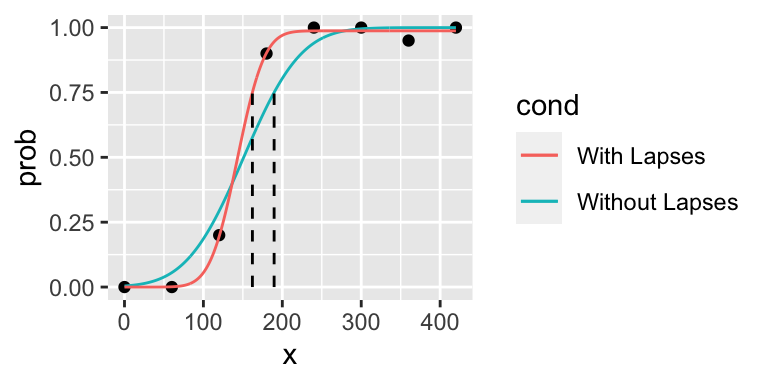## Allowing lapses to be free not always eliminate the bias Wichmann and Hill (2001) performed simulations using 7 sampling squemes defined as follows parweibull <- c(10, 3) create_xs <- function(i, f) tibble(squeme = i, y = f, x = inv_weibull_fun(f, parweibull)) s <- list() s[] <- create_xs(1, c(.3, .4, .48, .52, .6, .7)) s[] <- create_xs(2, c(.1, .3, .4, .6, .7, .9)) s[] <- create_xs(3, c(.3, .44, .7, .8, .9, .98)) s[] <- create_xs(4, c(.1, .2, .3, .4, .5, .6)) s[] <- create_xs(5, c(.08, .18, .28, .7, .85, .99)) s[] <- create_xs(6, c(.3, .4, .5, .6, .7, .99)) s[] <- create_xs(7, c(.34, .44, .54, .8, .9, .98)) s <- bind_rows(s) ggplot(s, aes(x = x, y = squeme, color = factor(squeme))) + geom_point() + geom_line() + labs(color = 'Sampling scheme') + theme(legend.position = 'top')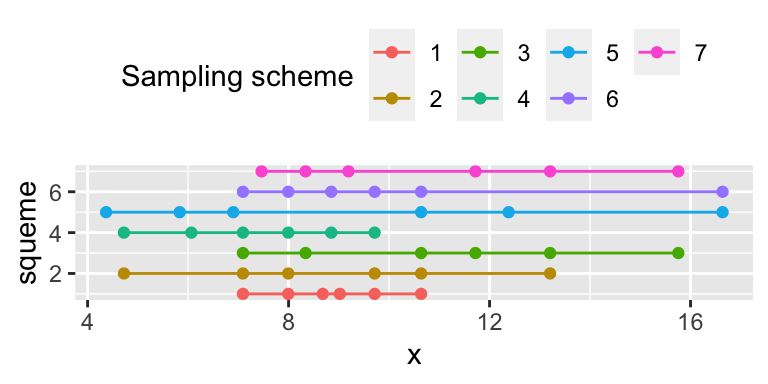Wichmann and Hill generated parametric bootstrap samples of data coming from a psychometric function with the shape of a weibull function with parameters $$10$$ and $$3$$, $$guess=0.5$$ and variable $$\lambda$$ (directly related to the lapses). For each value of the independent variable $$x$$, we simulated 160 trials (in the original paper 20, 40 and 80 trials were also used). library(tidyr) # to use crossing library(purrr) # to use map2 create_sim_dat <- function(d, l) { psychometric_fun <- create_psy_fun(weibull_fun, .5, l) ypred <- psychometric_fun(d$x, parweibull)
k <- rbinom(length(d$x), d$n, ypred)
tibble(x = d$x, k = k, n = d$n , y = k/d$n) } simdat <- crossing(s, n = 160, sample = 1:10, lambda = seq(0,.05, .01)) %>% group_by(squeme, sample, lambda) %>% nest() %>% mutate(d = map2(data, lambda, create_sim_dat)) %>% select(-data) %>% unnest(d) To illustrate the simulated data, we plot the first sample for each sampling scheme (columns) and each value of $$\lambda$$ (rows) p <- ggplot(simdat %>% filter(sample == 1)) + facet_grid(lambda ~ squeme) + geom_point(aes(x = x, y = y)) p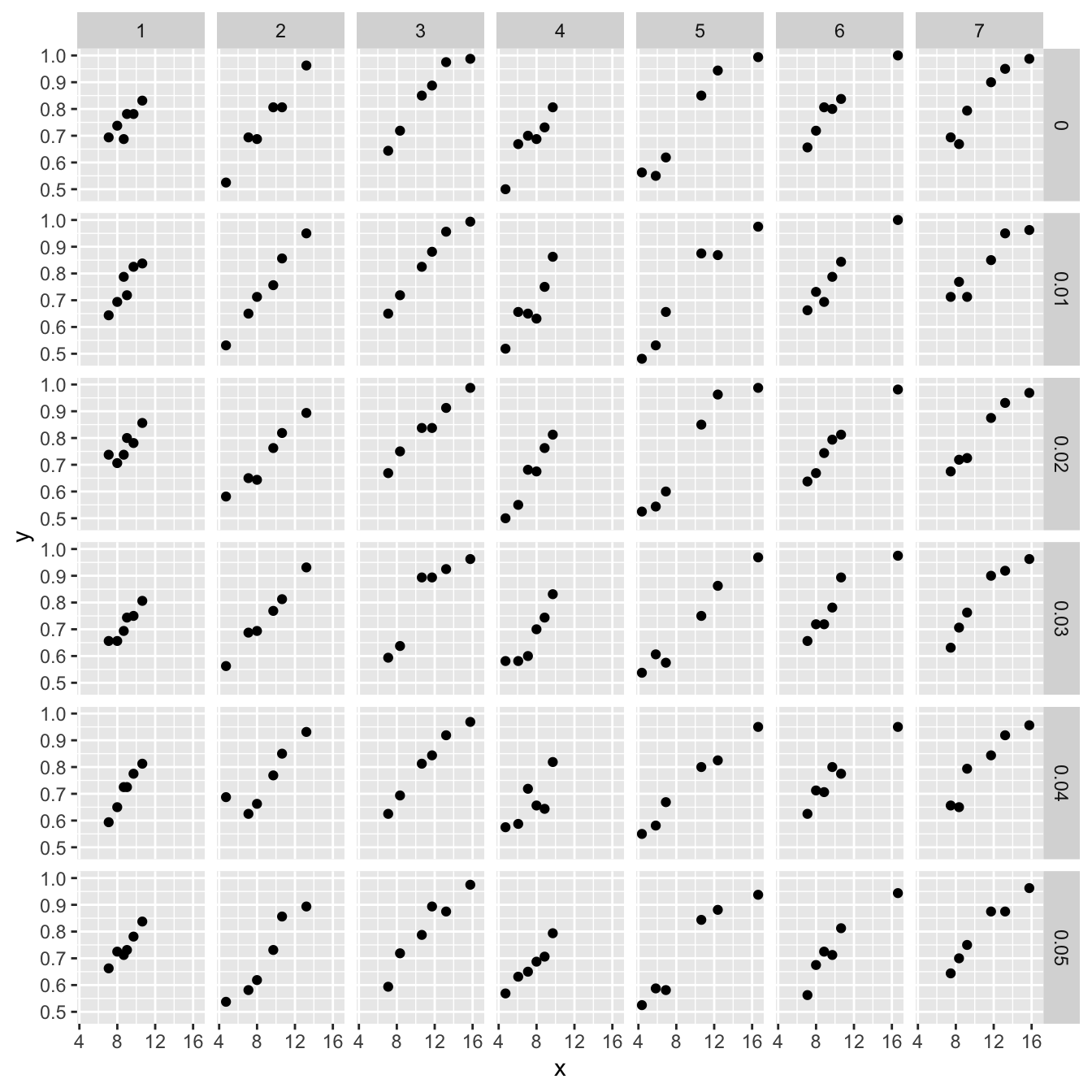Then, we use quickpsy to fit a weibull function to each condition using $$\lambda = 0$$ fit <- quickpsy(simdat, x, k, n, grouping = c("squeme", "lambda", "sample"), fun = weibull_fun, bootstrap = "none", guess = .5, lapses = 0, xmin = 4, xmax = 20)  and show the fitted psychometric function for the first sample p + geom_line(data = fit$curves %>% filter(sample == 1),
aes(x = x, y =y))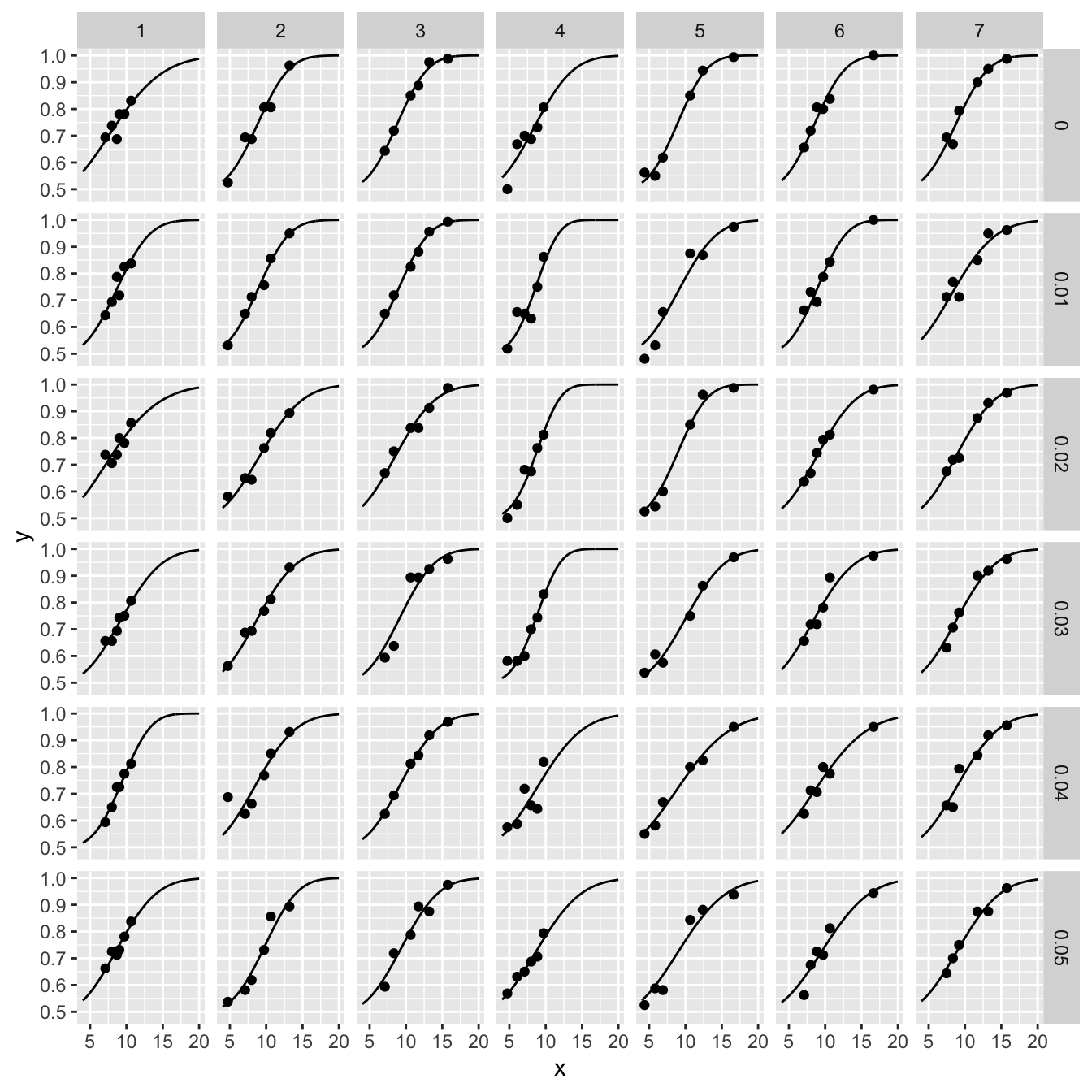Replicating Wichmann and Hill, we show that the thresholds are biased (overestimated) as $$\lambda$$ increases (they showed that the slope is also biased)

thre <- fit$thresholds %>% group_by(squeme, lambda) %>% summarise (threshold = mean(thre), .groups = "keep") real_threshold <- inv_weibull_fun((.75 - .5) / (1 - .5 - 0), parweibull) ggplot(thre) + geom_point(aes(x = lambda, y = threshold, color = factor(squeme))) + geom_hline(yintercept = real_threshold, lty = 2)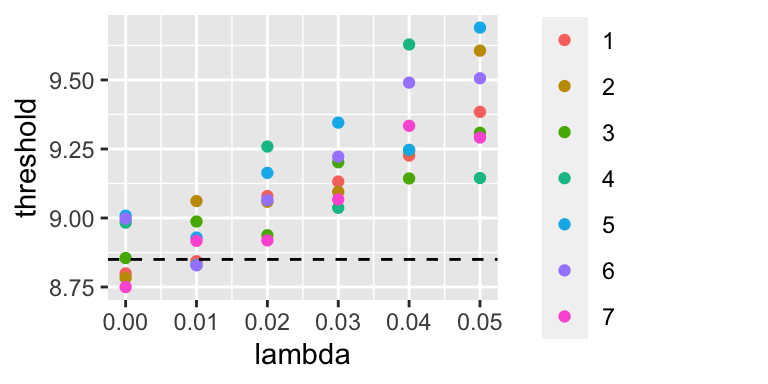Wichmann and Hill (2001) reported that allowing $$\lambda$$ to vary within a given small window eliminates the bias for the threshold, but consistently with Prins (2012) we were not able to replicate this finding fit_lapses <- quickpsy(simdat, x, k, n, grouping = c("squeme", "lambda", "sample"), fun = weibull_fun, bootstrap = "none", guess = .5, lapses = TRUE, xmin = 4, xmax = 20) thre_lapses <- fit_lapses$thresholds %>%
group_by(squeme, lambda) %>%
summarise(threshold = mean(thre, na.rm = TRUE), .groups = "keep") #

ggplot(thre_lapses) +
geom_point(aes(x = lambda, y = threshold, color = factor(squeme))) +
geom_hline(yintercept = real_threshold, lty = 2)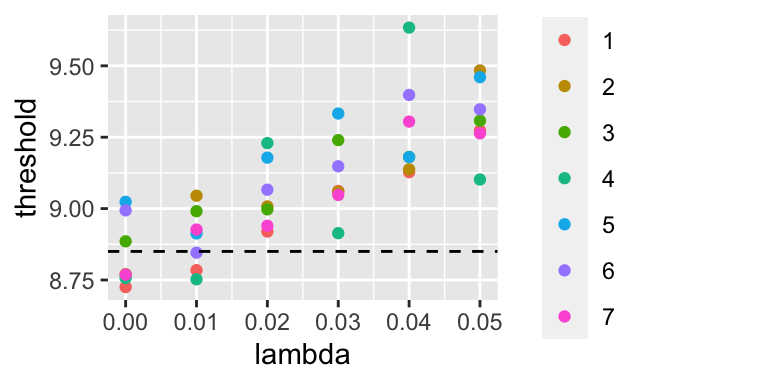This result indicates that fitting the lapses does not always eliminate the bias in threshold estimation.